## eBook ‘geometry and the absolute point’ v0.1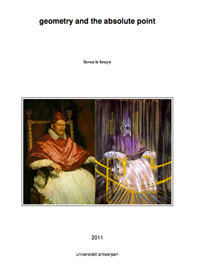In preparing for next year’s ‘seminar noncommutative geometry’ I’ve converted about 30 posts to LaTeX, centering loosely around the topics students have asked me to cover : noncommutative geometry, the absolute point (aka the field with one element), and their relation to the Riemann hypothesis.

The idea being to edit these posts thoroughly, add much more detail (and proofs) and also add some extra sections on Borger’s work and Witt rings (and possibly other stuff).

For those of you who prefer to (re)read these posts on paper or on a tablet rather than perusing this blog, you can now download the very first version (minimally edited) of the eBook ‘geometry and the absolute point’. All comments and suggestions are, of course, very welcome. I hope to post a more definite version by mid-september.

I’ve used the thesis-documentclass to keep the same look-and-feel of my other course-notes, but I would appreciate advice about turning LaTeX-files into ‘proper’ eBooks. I am aware of the fact that the memoir-class has an ebook option, and that one can use the geometry-package to control paper-sizes and margins.

Soon, I will be releasing a LaTeX-ed ‘eBook’ containing the Bourbaki-related posts. Later I might also try it on the games- and groups-related posts…

## mathblogging and poll-resultsMathblogging.org is a recent initiative and may well become the default starting place to check on the status of the mathematical blogosphere.

Handy, if you want to (re)populate your RSS-aggregator with interesting mathematical blogs, is their graphical presentation of (nearly) all math-blogs ordered by type : group blogs, individual researchers, teachers and educators, journalistic writers, communities, institutions and microblogging (twitter). Links to the last 7 posts are given so you can easily determine whether that particular blog is of interest to you.

The three people behind the project, Felix Breuer, Frederik von Heymann and Peter Krautzberger, welcome you to send them links to (micro)blogs they’ve missed. Surely, there must be a lot more mathematicians with a twitter-account than the few ones listed so far…

Even more convenient is their list of latest posts from their collection, ordered by date. I’ve put that page in my Bookmarks Bar the moment I discovered it! It would be nice, if they could provide an RSS-feed of this list, so that people could place it in their sidebar, replacing old-fashioned and useless blogrolls. The site does provide two feeds, but they are completely useless as they click through to empty pages…

While we’re on the topic of math-blogging, the results of the ‘What should we write about next?’-poll that ran the previous two days on the entry page. Of all people visiting that page, 2.6% left suggestions.

The vast majority (67%) wants more posts on noncommutative geometry. Most of you are craving for introductions (and motivation) accessible to undergraduates (as ‘it’s hard to find quality, updated information on this’). In particular, you want posts giving applications in mathematics (especially number theory), or explaining relationships between different approaches. One person knew exactly how I should go about to achieve the hoped-for accessibility : “As a rule, I’d take what you think would be just right for undergrads, and then trim it down a little more.”

Others want rather specialized posts, such as on ‘connection and parallel transport in noncommutative geometry’ or on ‘trees (per J-L. Loday, M. Aguiar, Connes/Kreimer renormalization (aka Butcher group)), or something completely other tree-related’.

Fortunately, some of you told me it was fine to write about ‘combinatorial games and cool nim stuff, finite simple groups, mathematical history, number theory, arithmetic geometry’, pushed me to go for ‘anything monstrous and moonshiney’ (as if I would know the secrets of the ‘connection between the Mathieu group M24 and the elliptic genus of K3’…) or wrote that ‘various algebraic geometry related posts are always welcome: posts like Mumford’s treasure map‘.

Published
Categorized as web

## How to play Nimbers?

Nimbers is a 2-person game, winnable only if you understand the arithmetic of the finite fields $\mathbb{F}_{2^{2^n}}$ associated to Fermat 2-powers.

It is played on a rectangular array (say a portion of a Go-board, for practical purposes) having a finite number of stones at distinct intersections. Here’s a typical position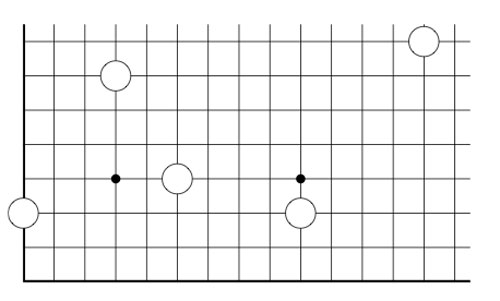The players alternate making a move, which is either

• removing one stone, or
• moving a stone to a spot on the same row (resp. the same column) strictly to the left (resp. strictly lower), and if there’s already a stone on this spot, both stones are removed, or
• adding stones to the empty corners of a rectangle having as its top-right hand corner a chosen stone and removing stones at the occupied corners

Here we illustrate two possible moves from the above position, in the first we add two new stones and remove 2 existing stones, in the second we add three new stones and remove only the top right-hand stone.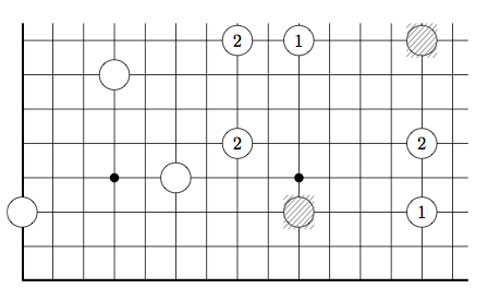As always, the last player able to move wins the game!

Note that Nimbers is equivalent to Lenstra’s ‘turning corners’-game (as introduced in his paper Nim-multiplication or mentioned in Winning Ways Chapter 14, page 473).

If all stones are placed on the left-most column (or on the bottom row) one quickly realizes that this game reduces to classical Nim with Nim-heap sizes corresponding to the stones (for example, the left-most stone corresponds to a heap of size 3).

Nim-addition $n \oplus m$ is defined inductively by

$n \oplus m = mex(n’ \oplus m,n \oplus m’)$

where $n’$ is any element of ${ 0,1,\ldots,n-1 }$ and $m’$ any element of ${ 0,1,\ldots,m-1 }$ and where ‘mex’ stands for Minimal EXcluded number, that is the smallest natural number which isn’t included in the set. Alternatively, one can compute $n \oplus m$ buy writing $n$ and $m$ in binary and add these binary numbers without carrying-over. It is well known that a winning strategy for Nim tries to shorten one Nim-heap such that the Nim-addition of the heap-sizes equals zero.

This allows us to play Nimber-endgames, that is, when all the stones have been moved to the left-column or the bottom row.

To evaluate general Nimber-positions it is best to add another row and column, the coordinate axes of the array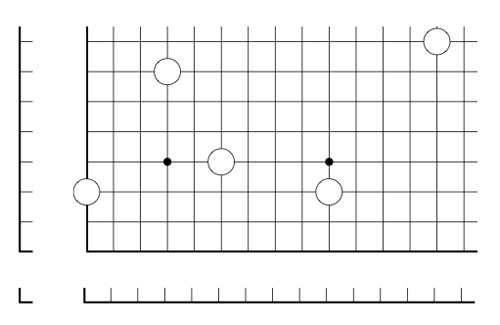and so our stones lie at positions (1,3), (4,7), (6,4), (10,3) and (14,8). In this way all legal moves follow the rectangle-rule when we allow rectangles to contain corners on the added coordinate axes. For example, removing a stone is achieved by taking a rectangle with two sides on the added axes, and, moving a stone to the left (or the bottom) is done by taking a rectangle with one side at the x-axes (resp. the y-axes)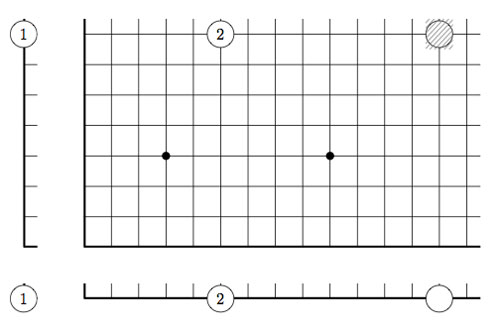However, the added stones on the coordinate axes are considered dead and may be removed from the game. This observation allows us to compute the Grundy number of a stone at position (m,n) to be

$G(m,n)=mex(G(m’,n’) \oplus G(m’,n) \oplus G(m,n’)~:~0 \leq m’ < m, 0 \leq n’ < n)$

and so by induction these Grundy numbers are equal to the Nim-multiplication $G(m,n) = m \otimes n$ where

$m \otimes n = mex(m’ \otimes n’ \oplus m’ \otimes n \oplus m \otimes n’~:~0 \leq m’ < m, 0 \leq n’ < n)$

Thus, we can evaluate any Nimbers-position with stone-coordinates smaller than $2^{2^n}$ by calculating in a finite field using the identification (as for example in the odd Knights of the round table-post) $\mathbb{F}_{2^{2^n}} = \{ 0,1,2,\ldots,2^{2^n}-1 \}$

For example, when all stones lie in a 15×15 grid (as in the example above), all calculations can be performed using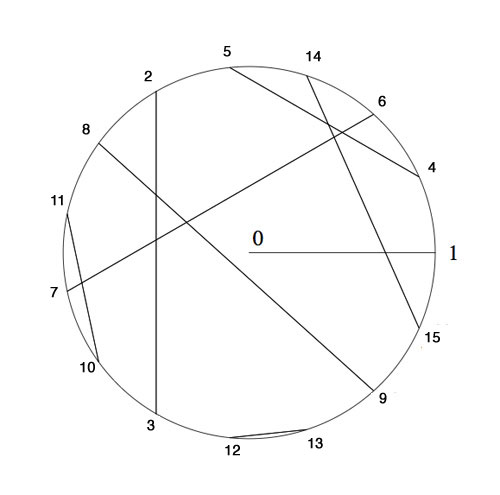Here, we’ve identified the non-zero elements of $\mathbb{F}_{16}$ with 15-th roots of unity, allowing us to multiply, and we’ve paired up couples $(n,n \oplus 1)$ allowing u to reduce nim-addition to nim-multiplication via

$n \oplus m = (n \otimes \frac{1}{m}) \otimes (m \oplus 1)$

In particular, the stone at position (14,8) is equivalent to a Nim-heap of size $14 \otimes 8=10$. The nim-value of the original position is equal to 8Suppose your opponent lets you add one extra stone along the diagonal if you allow her to start the game, where would you have to place it and be certain you will win the game?

Published
Categorized as games

## So, who did discover the Leech lattice?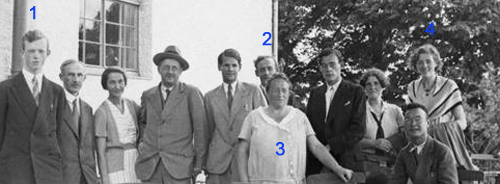For the better part of the 30ties, Ernst Witt (1) did hang out with the rest of the ‘Noetherknaben’, the group of young mathematicians around Emmy Noether (3) in Gottingen.

In 1934 Witt became Helmut Hasse‘s assistent in Gottingen, where he qualified as a university lecturer in 1936. By 1938 he has made enough of a name for himself to be offered a lecturer position in Hamburg and soon became an associate professor, the down-graded position held by Emil Artin (2) until he was forced to emigrate in 1937.

A former fellow student of him in Gottingen, Erna Bannow (4), had gone earlier to Hamburg to work with Artin. She continued her studies with Witt and finished her Ph.D. in 1939. In 1940 Erna Bannow and Witt married.

So, life was smiling on Ernst Witt that sunday january 28th 1940, both professionally and personally. There was just one cloud on the horizon, and a rather menacing one. He was called up by the Wehrmacht and knew he had to enter service in february. For all he knew, he was spending the last week-end with his future wife… (later in february 1940, Blaschke helped him to defer his military service by one year).

Still, he desperately wanted to finish his paper before entering the army, so he spend most of that week-end going through the final version and submitted it on monday, as the published paper shows.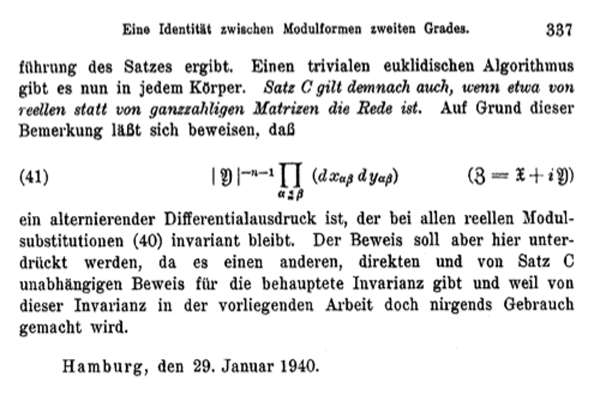In the 70ties, Witt suddenly claimed he did discover the Leech lattice ${\Lambda}$ that sunday. Last time we have seen that the only written evidence for Witt’s claim is one sentence in his 1941-paper Eine Identität zwischen Modulformen zweiten Grades. “Bei dem Versuch, eine Form aus einer solchen Klassen wirklich anzugeben, fand ich mehr als 10 verschiedene Klassen in ${\Gamma_{24}}$.”

But then, why didn’t Witt include more details of this sensational lattice in his paper?

Ina Kersten recalls on page 328 of Witt’s collected papers : “In his colloquium talk “Gitter und Mathieu-Gruppen” in Hamburg on January 27, 1970, Witt said that in 1938, he had found nine lattices in ${\Gamma_{24}}$ and that later on January 28, 1940, while studying the Steiner system ${S(5,8,24)}$, he had found two additional lattices ${M}$ and ${\Lambda}$ in ${\Gamma_{24}}$. He continued saying that he had then given up the tedious investigation of ${\Gamma_{24}}$ because of the surprisingly low contribution

$\displaystyle | Aut(\Lambda) |^{-1} < 10^{-18}$

to the Minkowski density and that he had consented himself with a short note on page 324 in his 1941 paper.”

In the last sentence he refers to the fact that the sum of the inverse orders of the automorphism groups of all even unimodular lattices of a given dimension is a fixed rational number, the Minkowski-Siegel mass constant. In dimension 24 this constant is

$\displaystyle \sum_{L} \frac{1}{| Aut(L) |} = \frac {1027637932586061520960267}{129477933340026851560636148613120000000} \approx 7.937 \times 10^{-15}$

That is, Witt was disappointed by the low contribution of the Leech lattice to the total constant and concluded that there might be thousands of new even 24-dimensional unimodular lattices out there, and dropped the problem.

If true, the story gets even better : not only claims Witt to have found the lattices ${A_1^{24}=M}$ and ${\Lambda}$, but also enough information on the Leech lattice in order to compute the order of its automorphism group ${Aut(\Lambda)}$, aka the Conway group ${Co_0 = .0}$ the dotto-group!

Is this possible? Well fortunately, the difficulties one encounters when trying to compute the order of the automorphism group of the Leech lattice from scratch, is one of the better documented mathematical stories around.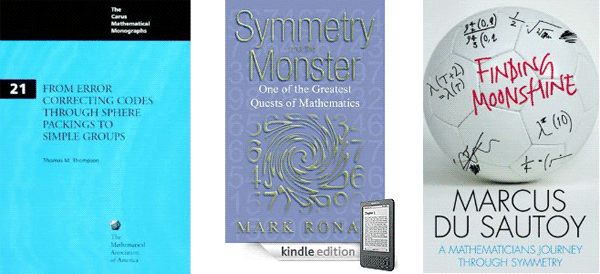The books From Error-Correcting Codes through Sphere Packings to Simple Groups by Thomas Thompson, Symmetry and the monster by Mark Ronan, and Finding moonshine by Marcus du Sautoy tell the story in minute detail.

It took John Conway 12 hours on a 1968 saturday in Cambridge to compute the order of the dotto group, using the knowledge of Leech and McKay on the properties of the Leech lattice and with considerable help offered by John Thompson via telephone.

But then, John Conway is one of the fastest mathematicians the world has known. The prologue of his book On numbers and games begins with : “Just over a quarter of a century ago, for seven consecutive days I sat down and typed from 8:30 am until midnight, with just an hour for lunch, and ever since have described this book as “having been written in a week”.”

Conway may have written a book in one week, Ernst Witt did complete his entire Ph.D. in just one week! In a letter of August 1933, his sister told her parents : “He did not have a thesis topic until July 1, and the thesis was to be submitted by July 7. He did not want to have a topic assigned to him, and when he finally had the idea, he started working day and night, and eventually managed to finish in time.”

So, if someone might have beaten John Conway in fast-computing the dottos order, it may very well have been Witt. Sadly enough, there is a lot of circumstantial evidence to make Witt’s claim highly unlikely.

For starters, psychology. Would you spend your last week-end together with your wife to be before going to war performing an horrendous calculation?

Secondly, mathematical breakthroughs often arise from newly found insight. At that time, Witt was also working on his paper on root lattices “Spiegelungsgrupen and Aufzähling halbeinfacher Liescher Ringe” which he eventually submitted in january 1941. Contained in that paper is what we know as Witt’s lemma which tells us that for any integral lattice the sublattice generated by vectors of norms 1 and 2 is a direct sum of root lattices.

This leads to the trick of trying to construct unimodular lattices by starting with a direct sum of root lattices and ‘adding glue’. Although this gluing-method was introduced by Kneser as late as 1967, Witt must have been aware of it as his 16-dimensional lattice ${D_{16}^+}$ is constructed this way.

If Witt wanted to construct new 24-dimensional even unimodular lattices in 1940, it would be natural for him to start off with direct sums of root lattices and trying to add vectors to them until he got what he was after. Now, all of the Niemeier-lattices are constructed this way, except for the Leech lattice!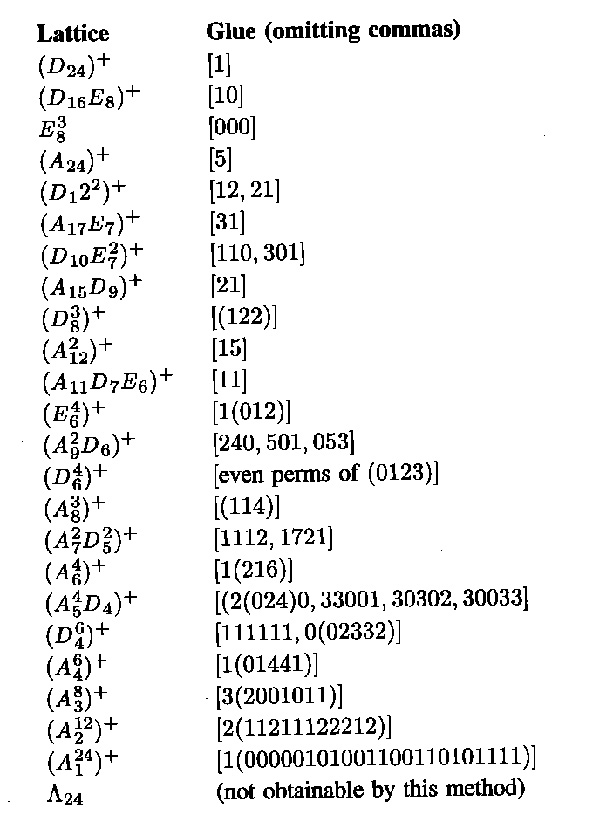I’m far from an expert on the Niemeier lattices but I would say that Witt definitely knew of the existence of ${D_{24}^+}$, ${E_8^3}$ and ${A_{24}^+}$ and that it is quite likely he also constructed ${(D_{16}E_8)^+, (D_{12}^2)^+, (A_{12}^2)^+, (D_8^3)^+}$ and possibly ${(A_{17}E_7)^+}$ and ${(A_{15}D_9)^+}$. I’d rate it far more likely Witt constructed another two such lattices on sunday january 28th 1940, rather than discovering the Leech lattice.

Finally, wouldn’t it be natural for him to include a remark, in his 1941 paper on root lattices, that not every even unimodular lattices can be obtained from sums of root lattices by adding glue, the Leech lattice being the minimal counter-example?

If it is true he was playing around with the Steiner systems that sunday, it would still be a pretty good story he discovered the lattices ${(A_2^{12})^+}$ and ${(A_1^{24})^+}$, for this would mean he discovered the Golay codes in the process!

Which brings us to our next question : who discovered the Golay code?

Published
Categorized as stories

## Seating the first few thousand Knights

The Knight-seating problems asks for a consistent placing of n-th Knight at an odd root of unity, compatible with the two different realizations of the algebraic closure of the field with two elements.
The first identifies the multiplicative group of its non-zero elements with the group of all odd complex roots of unity, under complex multiplication. The second uses Conway’s ‘simplicity rules’ to define an addition and multiplication on the set of all ordinal numbers.

The odd Knights of the round table-problem asks for a specific one-to-one correspondence between two realizations of ‘the’ algebraic closure $\overline{\mathbb{F}_2}$ of the field of two elements.

The first identifies the multiplicative group of its non-zero elements with the group of all odd complex roots of unity, under complex multiplication. The addition on $\overline{\mathbb{F}_2}$ is then recovered by inducing an involution on the odd roots, pairing the one corresponding to x to the one corresponding to x+1.

The second uses Conway’s ‘simplicity rules’ to define an addition and multiplication on the set of all ordinal numbers. Conway proves in ONAG that this becomes an algebraically closed field of characteristic two and that $\overline{\mathbb{F}_2}$ is the subfield of all ordinals smaller than $\omega^{\omega^{\omega}}$. The finite ordinals (the natural numbers) form the quadratic closure of $\mathbb{F}_2$.

On the natural numbers the Conway-addition is binary addition without carrying and Conway-multiplication is defined by the properties that two different Fermat-powers $N=2^{2^i}$ multiply as they do in the natural numbers, and, Fermat-powers square to its sesquimultiple, that is $N^2=\frac{3}{2}N$. Moreover, all natural numbers smaller than $N=2^{2^{i}}$ form a finite field $\mathbb{F}_{2^{2^i}}$. Using distributivity, one can write down a multiplication table for all 2-powers.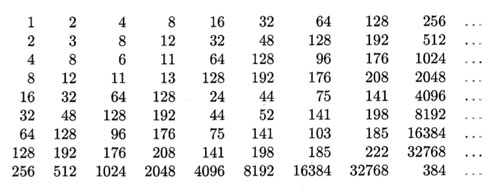The Knight-seating problems asks for a consistent placing of n-th Knight $K_n$ at an odd root of unity, compatible with the two different realizations of $\overline{\mathbb{F}_2}$. Last time, we were able to place the first 15 Knights as below, and asked where you would seat $K_{16}$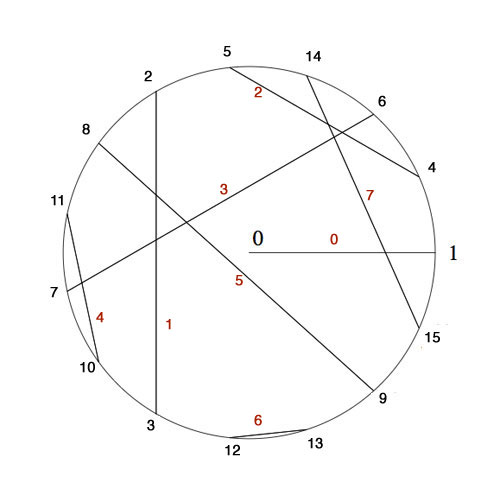$K_4$ was placed at $e^{2\pi i/15}$ as 4 was the smallest number generating the ‘Fermat’-field $\mathbb{F}_{2^{2^2}}$ (with multiplicative group of order 15) subject to the compatibility relation with the generator 2 of the smaller Fermat-field $\mathbb{F}_2$ (with group of order 15) that $4^5=2$.

To include the next Fermat-field $\mathbb{F}_{2^{2^3}}$ (with multiplicative group of order 255) consistently, we need to find the smallest number n generating the multiplicative group and satisfying the compatibility condition $n^{17}=4$. Let’s first concentrate on finding the smallest generator : as 2 is a generator for 1st Fermat-field $\mathbb{F}_{2^{2^1}}$ and 4 a generator for the 2-nd Fermat-field $\mathbb{F}_{2^{2^2}}$ a natural conjecture might be that 16 is a generator for the 3-rd Fermat-field $\mathbb{F}_{2^{2^3}}$ and, more generally, that $2^{2^i}$ would be a generator for the next field $\mathbb{F}_{2^{2^{i+1}}}$.

However, an “exercise” in the 1978-paper by Hendrik Lenstra Nim multiplication asks : “Prove that $2^{2^i}$ is a primitive root in the field $\mathbb{F}_{2^{2^{i+1}}}$ if and only if i=0 or 1.”

I’ve struggled with several of the ‘exercises’ in Lenstra’s paper to the extend I feared Alzheimer was setting in, only to find out, after taking pen and paper and spending a considerable amount of time calculating, that they are indeed merely exercises, when looked at properly… (Spoiler-warning : stop reading now if you want to go through this exercise yourself).

In the picture above I’ve added in red the number $x(x+1)=x^2+1$ to each of the involutions. Clearly, for each pair these numbers are all distinct and we see that for the indicated pairing they make up all numbers strictly less than 8.

By Conway’s simplicity rules (or by checking) the pair (16,17) gives the number 8. In other words, the equation
$x^2+x+8$ is an irreducible polynomial over $\mathbb{F}_{16}$ having as its roots in $\mathbb{F}_{256}$ the numbers 16 and 17. But then, 16 and 17 are conjugated under the Galois-involution (the Frobenius $y \mapsto y^{16}$). That is, we have $16^{16}=17$ and $17^{16}=16$ and hence $16^{17}=8$. Now, use the multiplication table in $\mathbb{F}_{16}$ given in the previous post (or compute!) to see that 8 is of order 5 (and NOT a generator). As a consequence, the multiplicative order of 16 is 5×17=85 and so 16 cannot be a generator in $\mathbb{F}_{256}$.
For general i one uses the fact that $2^{2^i}$ and $2^{2^i}+1$ are the roots of the polynomial $x^2+x+\prod_{j<i} 2^{2^j}$ over $\mathbb{F}_{2^{2^i}}$ and argues as before.

Right, but then what is the minimal generator satisfying $n^{17}=4$? By computing we see that the pairings of all numbers in the range 16…31 give us all numbers in the range 8…15 and by the above argument this implies that the 17-th powers of all numbers smaller than 32 must be different from 4. But then, the smallest candidate is 32 and one verifies that indeed $32^{17}=4$ (use the multiplication table given before).

Hence, we must place Knight $K_{32}$ at root $e^{2 \pi i/255}$ and place the other Knights prior to the 256-th at the corresponding power of 32. I forgot the argument I used to find-by-hand the requested place for Knight 16, but one can verify that $32^{171}=16$ so we seat $K_{16}$ at root $e^{342 \pi i/255}$.

But what about Knight $K_{256}$? Well, by this time I was quite good at squaring and binary representations of integers, but also rather tired, and decided to leave that task to the computer.

If we denote Nim-addition and multiplication by $\oplus$ and $\otimes$, then Conway’s simplicity results in ONAG establish a field-isomorphism between $~(\mathbb{N},\oplus,\otimes)$ and the field $\mathbb{F}_2(x_0,x_1,x_2,\ldots )$ where the $x_i$ satisfy the Artin-Schreier equations

$x_i^2+x_i+\prod_{j < i} x_j = 0$

and the i-th Fermat-field $\mathbb{F}_{2^{2^i}}$ corresponds to $\mathbb{F}_2(x_0,x_1,\ldots,x_{i-1})$. The correspondence between numbers and elements from these fields is given by taking $x_i \mapsto 2^{2^i}$. But then, wecan write every 2-power as a product of the $x_i$ and use the binary representation of numbers to perform all Nim-calculations with numbers in these fields.

Therefore, a quick and dirty way (and by no means the most efficient) to do Nim-calculations in the next Fermat-field consisting of all numbers smaller than 65536, is to use sage and set up the field $\mathbb{F}_2(x_0,x_1,x_2,x_3)$ by

R.< x,y,z,t > =GF(2)[]
S.< a,b,c,d >=R.quotient((x^2+x+1,y^2+y+x,z^2+z+x*y,t^2+t+x*y*z))


To find the smallest number generating the multiplicative group and satisfying the additional compatibility condition $n^{257}=32$ we have to find the smallest binary number $i_1i_2 \ldots i_{16}$ (larger than 255) satisfying

(i1*a*b*c*t+i2*b*c*t+i3*a*c*t+i4*c*t+i5*a*b*t+i6*b*t+
i7*a*t+i8*t+i9*a*b*c+i10*b*c+i11*a*c+i12*c+i13*a*b+
i14*b+i15*a+i16)^257=a*c


It takes a 2.4GHz 2Gb-RAM MacBook not that long to decide that the requested generator is 1051 (killing another optimistic conjecture that these generators might be 2-powers). So, we seat Knight
$K_{1051}$ at root $e^{2 \pi i/65535}$ and can then arrange seatings for all Knight queued up until we reach the 65536-th! In particular, the first Knight we couldn’t place before, that is Knight $K_{256}$, will be seated at root $e^{65826 \pi i/65535}$.

If you’re lucky enough to own a computer with more RAM, or have the patience to make the search more efficient and get the seating arrangement for the next Fermat-field, please drop a comment.

I’ll leave you with another Lenstra-exercise which shouldn’t be too difficult for you to solve now : “Prove that $x^3=2^{2^i}$ has three solutions in $\mathbb{N}$ for each $i \geq 2$.”